Technical Article

# How to Design a Fuzzy Logic Controller

September 29, 2021 by Sara McCaslin

## Let’s review how fuzzy logic and ladder logic work together, how to design a fuzzy logic controller, and some advantages and disadvantages of using fuzzy logic.

Fuzzy logic controllers are, quite literally, all around us. From anti-lock braking systems to the washing machines that clean our clothes. But do they really work? And what are the benefits of using them?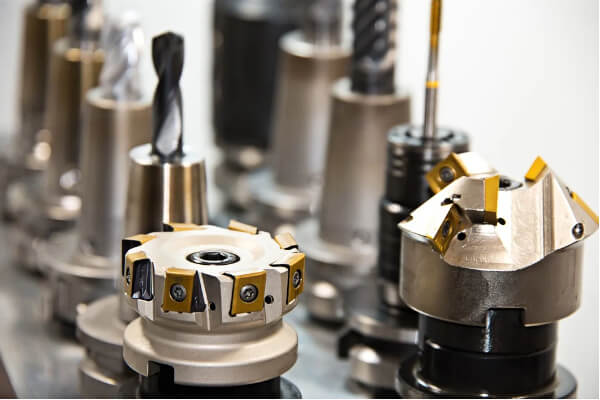### Ladder Logic and Fuzzy Logic

A good starting point in learning about fuzzy logic controls is determining if ladder logic is compatible with fuzzy logic. Ladder logic is a commonly used rule-based approach to programming PLCs (programmable logic controllers) when sequential logic is needed. Each of the rungs in a ladder logic program represents a logic equation that is either binary or discrete.

Keeping in mind that ladder logic is read from left to right, top to bottom, then the far right side of a rung represents the output condition. The output condition of that rung depends on the outcome of the ladder logic symbols to the left of the output.

Take, as a basic example, the single rung shown in figure 2. Notice that the A/C (air conditioning) is only active when the Switch is active, and either HighTemp or Humid is active.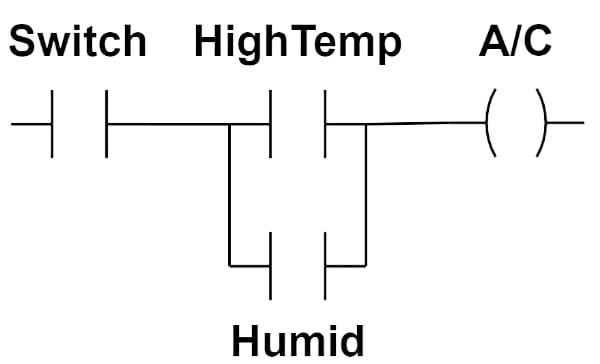##### Figure 2. Ladder logic rung for controlling an A/C system. Information used courtesy of Wikipedia

Expressed as a rule, this rung would take the following form:

A/C = Switch AND (HighTemp OR Humid)

Because ladder logic can be expressed as rules, it readily lends itself to adoption into fuzzy logic control.  Another way to think of this is that the outcome depends on the IF conditions represented by the ladder logic symbols to the left while the output represents the THEN result.

IF (Switch AND [HighTemp OR Humid]) THEN A/C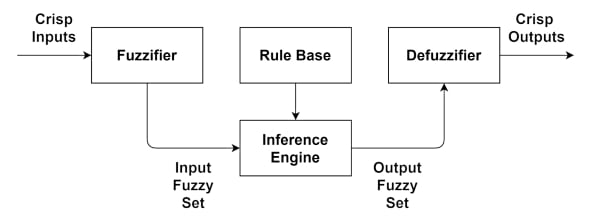##### Figure 3. Block diagram representing a fuzzy logic system.

This approach can certainly be implemented using fuzzy logic rules. In such a case, the crisp inputs would be temperature and relative humidity. Sensor reading of these values would be transformed into fuzzy inputs by a fuzzifier. The inference engine would contain, among other rules, the one shown above. Based on the rules of the inference engine, a fuzzy output set would be presented to the defuzzifier. From there, crisp outputs would be provided to the control system.

In short, ladder logic can be implemented using fuzzy logic.

### Basic Steps to Designing a Fuzzy Logic Controller

Fuzzy logic control systems (often abbreviated FLC) are an alternate approach to developing and implementing control systems.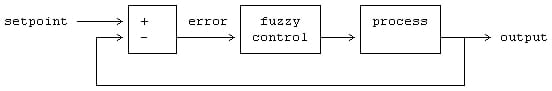#### Recognize the Assumptions Involved

Before beginning the process of designing an FLC, there are certain key assumptions.

• A solution exists to the control problem.
• A “good enough” solution is acceptable in place of an “optimal solution.”
• Input, output, and state variables can be observed and used for controlling.
• A body of knowledge exists in the form of:
• Linguistic rules, and/or
• A set of input-output data from which rules can be extracted.
• The FLC must be designed to achieve an acceptable range of precision.
• Stability and optimality are not addressed explicitly but are open.

#### Identify and Configure the Variables for Fuzzification

The first step in designing an FLC is identifying the input, output, and state variables involved. Next, determine the fuzzy subsets for the variables. For example, temperature input may be divided into five subsets with a descriptive linguistic label for each: {‘cold,’ ’cool,’ ‘nominal,’ ’warm,’ ’hot’}.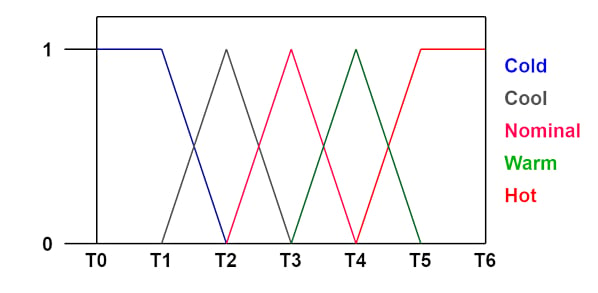##### Figure 5. Example of using membership functions to represent temperature.

Once the subsets have been chosen for the variables, membership functions should be configured to represent these subsets. Options for membership functions include triangular, trapezoidal, sigmoid, and Gaussian. This constitutes the fuzzifier that converts the crisp inputs into fuzzy values. Note that fuzzy subsets and associated membership functions also need to be configured for the output of the inference engine.

#### Generate the Fuzzy Logic Rules

Converting a traditional ladder logic controller into a fuzzy logic controller begins with generating fuzzy logic rules.

Alluded in the assumptions, this may take the form of linguistic rules that already exist or can be developed with the aid of a subject matter expert. Rules can also be generated given a set of input-output data. And, in the case of ladder logic, the fuzzy logic rules can be read directly from the ladder diagram.

The result is the rule base for the fuzzy system.

#### Implementing the Inference Engine and Defuzzifier

There are several different approaches to implementing the inference engine. In controls, the two most popular approaches are Mamdani and Sugeno. Both types of inference engines combine the fuzzy output from the rule base using an OR operator and subsequently defuzzifies that output to obtain a crisp value.

### Applications of Fuzzy Logic Controllers

Fuzzy logic controllers have found extremely widespread usage. For example, elevators often have fuzzy logic controllers that decrease wait time, minimize travel between floors, and reduce energy usage. An added benefit to minimizing travel between floors is reduced wear on critical components and longer service life.

Another fascinating example of fuzzy control systems involves digital video cameras and camcorders. Operators may move or shake the camera while filming a consistent shot, but fuzzy logic control can automatically compensate.

In an industrial setting, fuzzy logic is often used to control industrial processes and systems. For example, fuzzy logic works well with temperature and process controllers, such as those used with heat treatment of metals. Typical heat treatments involve highly precise ramp and soak cycles to achieve the correct metallurgical properties.

Similar applications that benefit from FLC include curing rubber or drying solvents on painted surfaces. In addition, all of these are examples of control processes that typically utilize a PID (proportional integral derivative) controller. CNC (computer numeric control) machining often depends on fuzzy logic controllers, as do many autonomous robotics, machine vision, and remote sensing.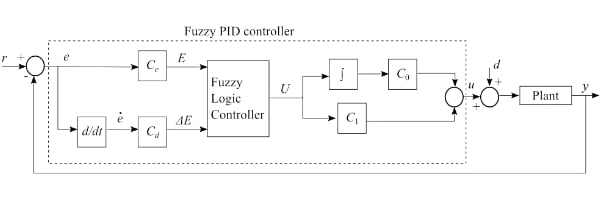##### Figure 6. Block diagram for a Fuzzy PID controller. Image used courtesy of Mathworks

Fuzzy logic control can still function when faced with imprecise data as well as non-linearity in the system. It also allows human experience and knowledge to be integrated into a control system and is highly customizable. Fuzzy logic control systems are generally cheaper to develop compared to more traditional approaches, and tend to be more efficient, robust, and reliable.

Specifically, in controls, FLC does not require the model and/or objective to be formulated in precise terms as is required in a more traditional approach to control systems. Furthermore, fuzzy controls can easily be blended with other control systems.

There are, of course, drawbacks to using fuzzy control systems. While fuzzy logic is a subset of artificial intelligence (AI), it is not a type of machine learning and cannot, therefore, adapt and learn. There are adaptive fuzzy logic controllers, but their design and complexity go well beyond the type of fuzzy controller under discussion.

In addition, updates will eventually need to be made to the rules that the controller depends on, and fuzzy logic controllers require extensive testing and validation before they are considered ready for implementation.

When should a fuzzy logic controller be used? They are an excellent option for control problems that are extremely complex and difficult to represent using precise models and objectives. They work well when imprecise data and nonlinear behavior are involved. They are also a good choice when human experience and expertise can be contributed to the system. How do you use fuzzy logic in your control system? Or, do you only use PID controllers?•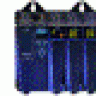pnachtwey October 01, 2021
•maddiebradshaw October 19, 2021